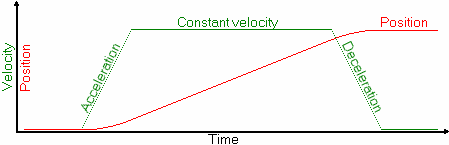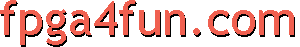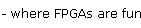## CNC 2 - Stepper control

##### Parallel interface

Here's a typical hobbyist CNC setup.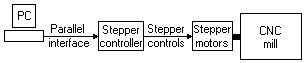A PC uses its parallel (printer) interface to connect to the stepper controller, which in turn drives the stepper motors (sometimes simply called "steppers") of the CNC mill.

Assuming our mill has three stepper motors, let's zoom in to see more details.The PC sends two signals per stepper motor (Step & Dir).
Then the stepper controller generates the stepper outputs (4 to 8 wires per stepper, depending on their types).

The PC must be dedicated to the CNC task because the timing of the Step/Dir signals must be as precise as possible. The PC must either run in DOS mode, or in Windows/Linux but with all other tasks shut down.

##### Step/Dir signals

The Step and Dir signals are easy to understand. Each Step pulse equals one step of a stepper motor, and Dir controls the direction. For example, let's move 4 steps clockwise, and then 4 steps counter-clockwise.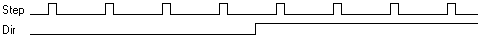Now the timing of these signals needs to be controlled.

##### Step/Dir timing

The stepper controller requires a minimum step pulse width (typically 1µs).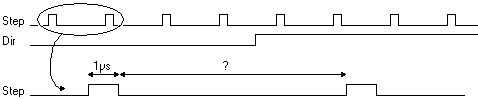But what is more important is the spacing of the pulses (the pulse rate per second) because that's what controls the motor speed.

##### Speed and acceleration

Let's assume your motor has 200 steps/turn, and that it is connected to an axis that requires 1 turn/mm. Also the stepper controller is configured in 16 micro-step mode (micro-stepping is used to make the steps smoother, and avoid vibrations in the mill).

So for each millimeter, we need 200x16x1=3200 steps. If we want to move at a speed of 100mm/s, we need 320000 steps/second. That means the steps needs to be separated by 1/320000 = 3.125µs.

Now like any mechanical system, we cannot reach a fixed speed instantaneously. So if we were at a still position and then started sending pulses separated by 3.125µs, the steppers wouldn't be happy (they would miss steps).

What we need is a smooth acceleration, to go from speed 0mm/s to speed 100mm/s.

##### Speed ramp

A common speed ramp is the trapezoidal profile.The ramp has three parts:

• Acceleration: A positive constant acceleration is applied
• Constant velocity: Acceleration is null.
• Deceleration: A negative constant acceleration is applied.

If we also draw the position, here's what we get.